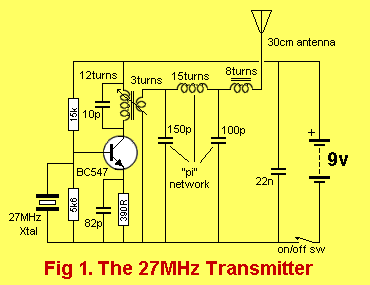Electronics-Lab.com Community

# What the value of Zout???

## Recommended Posts

Hello guys
The attached photo is a crystal oscillator
it is a voltage divider config. but replacing the RC with LC tuning tank
I need to know the out put impedance Zout??
Is it XC// XL at the operation freq 27MHz???

http://NOTE: sorry L= 3.48 uH not 1.2 uH as in the circuit##### Share on other sites

I didn't calculate the resonant frequency of the tank but if it is 27mHz then it is an extremely high impedance. Then the circuit will not start oscillating or it will stop oscillating if it has a load.

##### Share on other sites

thank you guru

but if it is 27mHz then it is an extremely high impedance
Be sure that the resonance freq is 27MHz
is the Zout = XC//XL; the parallel equivalent of the two reactancess (coil and ca)???
regards
##### Share on other sites

Yes, the output impedance will be equal to the impedance of the inductor and capacitor in parallel.

The impedance of an ideal inductor and capacitor in parallel is infinite, in reality is won't be infinite but it'll be very high and depend on the Q of the tank. Any load connected to the output will reduce the amplitude and stability of the oscillator because it will affect the Q of the resonant circuit.

##### Share on other sites

Any load connected to the output will reduce the amplitude and stability of the oscillator because it will affect the Q of the resonant circuit

What the solution then??
Do I have to replace the coil with a stepdown RF transfrmer??
What is the turn ratio then if i want to match with 100K ohm load?
##### Share on other sites

A 100k load is a high impedance to the LC parallel tuned circuit and will be fine. A 1k load would be much too low.

##### Share on other sites

If you use an output transformer to increase the load impedance then it steps down the signal voltage.
An emitter-follower transistor will increase the load impedance without the signal voltage loss.

##### Share on other sites

Hi Guru
Thank you for attention
What do you think of this circuitThe author use a transformer then PI network filter for impedance matching the low Z antenna (50 ohm) to the very high (near infinity) Z tank circuit.
I am a week ago, trying to understand, and I hope that you help me to understand
Thanks

##### Share on other sites

An alternative to building a transformer is to simply put a tap in the middle of the inductor, the apparent impedance of the load will be increased by a factor of four and the voltage across it will be halved so the power will be down by a factor of four.

##### Share on other sites

Hello guys
http://www.electronics-tutorials.com/amplifiers/negative-feedback.htm
the author speaks about how to made the necessary calculations to determine the transformer's turns ratio and also the LC needed for impedance matching a 50-ohm antenna to the preceding stage
Regardless of some of the details that the writer is talking about, espicially his talking about the dc biasing and the o/p voltage swing, I will focus in the discussion with you on the method of calculating the turns ratio.
Look at the fig:the author said:Here we are only mainly concerned here with the principle of a tuned circuit as a load. In this example we have a final load of 50 ohms connected to the output link coupling of our tuned circuit. Assume, just for discussion purposes, that in this example we needed a power output of 100 milliwatt from the amplifier and that we have available a power supply of 12V. Further,

Now:

2 * Po = [Vcc - Ve]^2 / R

or [2* 0.1] = [12 - 3.22]^2 / R

or [2* 0.1] = [ 8.78 ]^2 / R

and R = 77 / 0.2

= 385 ohms

Therefore the load presented to the output of our amplifier needs to be 385 ohms. The impedance ratio is 385 / 50 or 7.7:1 and the turns ratio on the transformer (inductor) is the square root of that number or 2.775:1.

My questions are:
1)Are these calculations are true in general?
2) Why '2' in 2.Po = [Vcc-Ve]^2/R, i learn that P = V^2/R
3)Why [Vcc-Ve]^2, I think it must be [Vcc-Vc]^2

Thanks

##### Share on other sites

• 2 weeks later...

My questions are:
1)Are these calculations are true in general?
2) Why '2' in 2.Po = [Vcc-Ve]^2/R, i learn that P = V^2/R
3)Why [Vcc-Ve]^2, I think it must be [Vcc-Vc]^2

Thanks

I discover the answer of the second question: the RMS value of the peak voltage = Vpeak/squre root of 2
and when we squre that root we get 2 which appear in the equation.

i need answer to question 3
thank you very much
##### Share on other sites

Hi walid,
I have not done RF work in my career and I haven't done school-work for about 42 years.

The transistor is a linear amplifier so its collector swings down to about 4.5V and swings up to the same amount above the supply voltage because of the tuned circuit. So the voltage swing is doubled.

Read further in the article where the author explains that the transistor is clipping and that its current should be higher. Then re-calculate the impedance of the transformer's primary.

## Join the conversation

You can post now and register later. If you have an account, sign in now to post with your account.×   Pasted as rich text.   Paste as plain text instead

Only 75 emoji are allowed.

×   Your previous content has been restored.   Clear editor

×   You cannot paste images directly. Upload or insert images from URL.